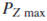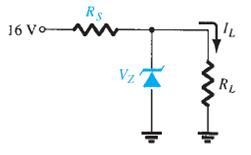Problem

# a. Design the network of Fig. to maintain VL at 12 V for a load variation (IL) from 0 mA t...

a. Design the network of Fig. to maintain VL at 12 V for a load variation (IL) from 0 mA to 200 mA. That is, determine RS and VZ.

b. Determinefor the Zener diode of part (a).

FIG.#### Step-by-Step Solution

Solution 1

Refer to Figure $$2.187$$ in the text book.

Calculate the Zener voltage.

\begin{aligned} V_{Z} &=V_{L} \\ &=12 \mathrm{~V} \end{aligned}

Therefore, the value of the Zener voltage is $$12 \mathrm{~V}$$.

Calculate the load resistance $$R_{L}$$.

$$R_{L}=\frac{V_{Z}}{I_{L}}$$

Here,

Zener voltage $$V_{Z}$$ is $$12 \mathrm{~V}$$

Load current $$I_{L}$$ is $$200 \mathrm{~mA}$$

Substitute $$12 \mathrm{~V}$$ for $$V_{Z}$$ and $$200 \mathrm{~mA}$$ for $$I_{L}$$ to obtain $$R_{L}$$.

\begin{aligned} R_{L} &=\frac{V_{Z}}{I_{L}} \\ &=\frac{12 \mathrm{~V}}{200 \mathrm{~mA}} \\ &=60 \Omega \end{aligned}

Calculate the source resistance $$R_{s}$$.

$$V_{L}=\frac{R_{L} V_{i}}{R_{L}+R_{S}}$$

Here,

Input voltage $$V_{i}$$ is $$16 \mathrm{~V}$$

Load voltage $$V_{L}$$ is $$12 \mathrm{~V}$$

Substitute $$16 \mathrm{~V}$$ for $$V_{i}, 60 \Omega$$ for $$R_{L}$$ and $$12 \mathrm{~V}$$ for $$V_{L}$$ to obtain $$R_{s}$$.

$$12=\frac{(60)(16)}{60+R_{s}}$$

$$720+12 R_{S}=960$$

$$12 R_{S}=240$$

$$R_{S}=20 \Omega$$

Therefore, the value of the source resistance $$R_{S}$$ is $$20 \Omega$$.

(b)

Calculate the maximum Zener diode power $$P_{z_{\max }}$$.

\begin{aligned} P_{Z_{\operatorname{man}}} &=V_{Z} I_{Z_{\max }} \\ &=(12 \mathrm{~V})(200 \mathrm{~mA}) \\ &=2.4 \mathrm{~W} \end{aligned}

Therefore, the maximum Zener diode power $$P_{Z_{\text {eax }}}$$ is $$2.4 \mathrm{~W}$$.

Add your Solution
Textbook Solutions and Answers Search
Solutions For Problems in Chapter 2